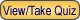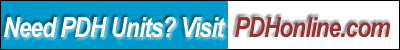Print this page

Basic Electrical Theory & Fundamentals

A. Bhatia, B.E.

Course Outline

Do you know where electricity comes from? To be able to say yes to that question, you must understand a bit about the physics of matter. In this course you'll learn the basics involving: matter, electron theory, magnetism, electromagnetism, the laws of electrical circuits, series circuits, parallel circuits, series-parallel circuits and the basic electrical formulas.

This 4-hr course material is based entirely on US Department of Energy training materials DOE-HDBK-1011/1-92, Fundamentals Handbook, Electrical Science, Volume 1 of 4, modules 1 and 2. The volumes 2, 3 and 4 of the handbook have been separately listed

This course includes a multiple-choice quiz at the end, which is designed to enhance the understanding of the course materials.

Learning Objective

At the conclusion of this course, the student will:

• Describe the electrostatic force, potential difference, electromotive force, ion charge etc.;
• Define the terminology such as conductor, insulator, resistor, electron current flow, direct current, alternating current, ideal source and real source;
• Understand the Ohm's law -the fundamental equation;
• Define the terms such as magnetic flux, permeability, ampere turns, field intensity, reluctance etc.;
• Describe how the ferromagnetic, paramagnetic and diamagnetic materials relate to permeability;
• Describe the physical qualities of a simple magnetic circuit;
• Describe the shape and components of BH magnetization curve;
• Understand the Faraday's and Lenz's law of induction;
• Understand the electrical symbology;
• Describe the half wave and full wave bridge rectifier;
• Distinguish between schematic diagram, one-line diagram, block diagram and wiring diagram;
• Distinguish between open, closed and short circuits;
• Be able to calculated total resistance for a series or parallel circuit;
• Understand the Kirchhoff's voltage and current law; and
• Describe the voltage and current effects in a open and shorted DC circuits:

Intended Audience

This course is aimed at beginners, novice engineers, electricians, hobbyists, plant mechanics, service technicians, maintenance supervisors, plant engineers, contractors, energy auditors, layout professionals and general audience.

Course Introduction

Imagine what life could be without electricity. Many people take for granted what we have today, and it is easy to forget how much we depend on it. Today we can play video games, use computers, watch television, listen to the radio, or talk on the phone. Control over the environment, refrigerating & microwave your food, instantaneous communications….. practically everything works on electricity. The understanding of electrical theory is necessary for almost every aspect of life. Simply stating electricity is the transfer of electrons (negative charges) from one place to another and the basis of understanding how electricity works is Ohm's law.

In this course, you are required to study the following DOE-HDBK-1011/1-92, Fundamentals Handbook, Electrical Science, Volume 1 of 4, modules 1 and 2.

Course Content

This course is based entirely on US Department of Energy training materials DOE-HDBK-1011/1-92, Fundamentals Handbook, Electrical Science, Volume 1 of 4, modules 1 and 2.

The link to the document is Basic Electrical Theory & Fundamentals.

Course Summary

Remember these facts:

1) Voltage is the pressure or the Electromotive Force that will push or pull electrons
2) Amperage is the number of electrons moving through a wire or conductor
3) Resistance will restrict how many amps can flow through a conductor
4) Increasing the electrical pressure will cause more amps to flow
5) Increasing the resistance will cause less amps to flow
6) The same current flows through each part of a series circuit.
7) Total Resistance of a series circuit is equal to the sum of the individual resistances.
8) The total voltage across a series circuit is equal to the sum of the individual voltage drops.
9) The voltage drop across a resistor in a series circuit is proportional to the size of the resistor.
10) The total power dissipated in a series circuit is equal to the sum of the individual power dissipations.
11) The same voltage exists across each branch of a parallel circuit and is equal to the source voltage.
12) The current through a branch of a parallel network is inversely proportional to the amount of resistance of the branch.
13) The total current of a parallel circuit is equal to the sum of the currents of the individual branches of the circuit.
14) The total resistance of a parallel circuit is equal to the reciprocal of the sum of the reciprocals of the individual resistances of the circuit.
15) The total power dissipated in a parallel circuit is equal to the sum of the individual power dissipations.
16) The total resistance of resistors in parallel is always less than the value of any one resistor
17) The total resistance of parallel resistors that are all the same value is that value divided by the number of resistors.
18) Always use the product over sum rule to break down two parallel resistors into one resistor. This is much easier than trying to solve large algebraic expressions.
19) The sum of the voltage drops around a closed loop is equal to the sum of the voltage sources of that loop (Kirchhoff's Voltage Law)
20) The current arriving at any junction point in a circuit is equal to the current leaving that junction (Kirchhoff's Current Law)

Quiz

Once you finish studying the above course content, you need to take a quiz to obtain the PDH credits.DISCLAIMER: The materials contained in the online course are not intended as a representation or warranty on the part of PDH Center or any other person/organization named herein. The materials are for general information only. They are not a substitute for competent professional advice. Application of this information to a specific project should be reviewed by a registered architect and/or professional engineer/surveyor. Anyone making use of the information set forth herein does so at their own risk and assumes any and all resulting liability arising therefrom.